GFG App
Open AppBrowser
Continue

# How does the Google’s “Did you mean” Algorithm work?

When you wish to say something but miss a few characters/words, the first response that anyone gives you is “Did you mean this?“. The same is the case with Google’s search algorithms. As soon as you search for a misspelled word or term on Google, it shows you the famous “Did you mean” alternative. We are sure you have come across a such scenario at least once. But have you ever wondered how Google figures it out? How does this Google’s Did you mean algorithm work?

To answer all your such queries, in this blog, we have covered a detailed analysis of how Google’s Did you mean algorithm works, what goes behind each step of the autocorrect feature of Google’s Did you mean algorithm and more.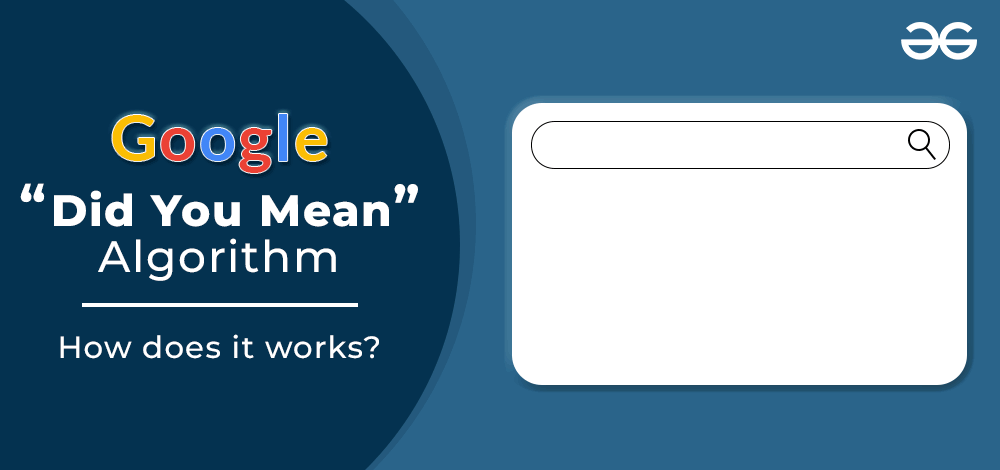How does the Google “Did you mean?” Algorithm work?

## Google’s Did you mean Algorithm:

Now that we have understood what is Google’s Did you mean feature, it’s time to discover the algorithm behind it. There are substantially 5 steps involved in the successful working of the Did you mean algorithm, as shown in the image below:

Let us look into these steps one by one in detail.

## 1. Finding possible substitute list using K-Gram and Jaccard Distance:

A k-gram index maps a k-gram to a posting list of all possible vocabulary terms that contain it. In this step, the possible list of alternatives/substitutes is found using the Jaccard Distance.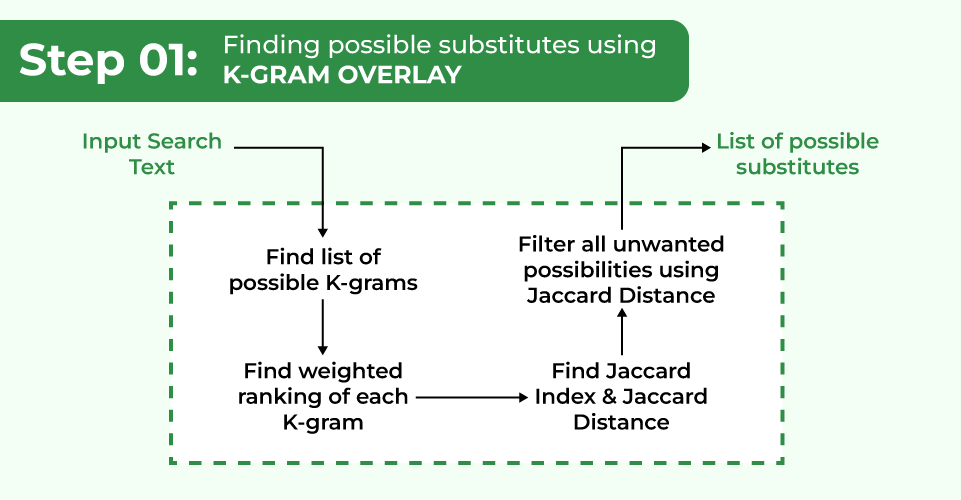Finding possible substitutes using K-Gram

For example, the figure below shows the k-gram postings list corresponding to the bigram “ur”. It is noteworthy that the postings list is sorted alphabetically.

For input “ur”   ->  The possible posting list will contain:

• blurry
• burn
• cure
• curfew
• surf
• yogurt
• yourself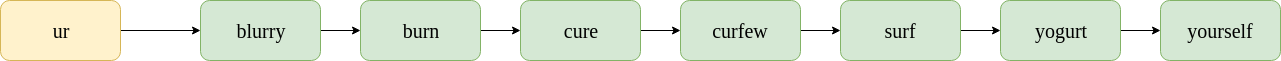The bigram of “ur”

### How Spelling Correction occurs using K-Gram Index and Jaccard Distance?

While creating the candidate list of possible corrected words, we can use the “k-gram overlap” to find the most likely corrections.

Consider the misspelled word: “appe”. The posting lists for the bigrams contained in it are shown below. Note that these are only sample subsets of the postings lists. the actual postings list would, of course, contain thousands of words in them.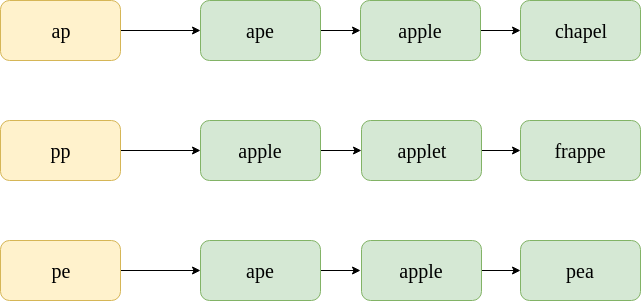The bigrams of word “appe.

To find the k-gram overlap between two postings lists, we use the Jaccard coefficient. Here, A and B are two sets (posting lists), A for the misspelled word and B for the corrected word.

The Jaccard Distance between the two sets can be calculated by using the formula:Jaccard Distance

For Word: “ape” :

To find the Jaccard coefficient, simply scan through the postings lists of all bigrams of “appe” and count the instances where “ape” appears.
In the first postings list, “ape” appears 1 time. In the second postings list, “ape” appears 0 times. In the third postings list, “ape” appears 1 time. Therefore, A ∩ B = 2. Now, the no. of bigrams in “appe” is 3, and the no. of bigrams in “ape” is 2. Therefore, A ∩ B = 3+2-2 = 3.

J(A, B) = 2/3 = 0.67.

For Word:“apple” :

A ∩ B = 3. Now, the no. of bigrams in “appe” is 3, and the no. of bigrams in “apple” is 4. Therefore, A ∩ B = 3+4-3 = 4.

J(A, B) = 3/4 = 0.75.

This suggests that “apple” is a more plausible correction. Practically, this method is used to filter out unlikely corrections.

Hence, the search results are then ranked based on a weight ranking function, and keywords with a Jaccard coefficient less than a predetermined threshold value are automatically eliminated.

## 2. Filter unwanted substitutes using Levenshtein Distance:

The Levenshtein distance is also referred to as the Edit Distance. Levenshtein distance calculates the distance between two words and returns a number representing how similar they are. The lower the distance (i.e. the smaller the number returned), the more similar they are.

Operations available in Levenshtein distance are:

1. Insertion: Insert a character at a position (cost: 1)
2. Deletion: Delete a character at any position (cost: 1)
3. Replace: Replace a character at any position with another character (cost: 1)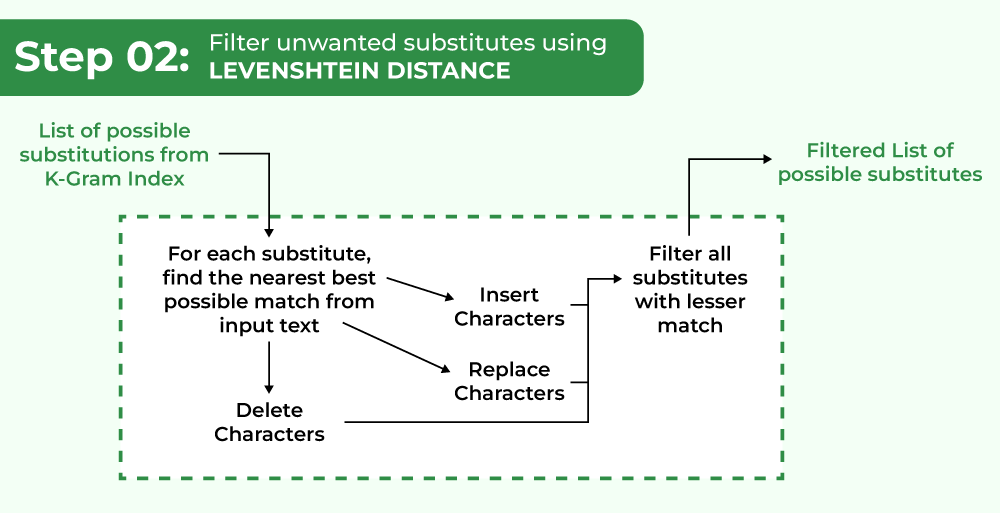Filter unwanted substitutes using Levenshtein Distance

Example:

The Levenshtein distance between “kitten” and “sitting” is 3, because the only 3 edits are required to change one word into the other, and there is no way to do it with fewer than 3 edits:

Steps to change kitten –> sitting:

1. kitten → sitten (substitution of “s” for “k”),
2. sitten → sittin (substitution of “i” for “e”),
3. sittin → sitting (insertion of “g” at the end).

## 3. Filtering substitutes based on cost using Damerau–Levenshtein distance:

Damerau Levenshtein distance is a variant of Levenshtein distance which is a type of Edit distance. Edit distance is a large class of distance metric for measuring the dissimilarity between two strings by computing a minimum number of operations (from a set of operations) used to convert one string to another string. It can be seen as a way of pairwise string alignment.

Damerau came up with his automated solution after an investigation showed that over 80% of the errors were because of:

1. A single deletion,
2. Insertion
3. Substitution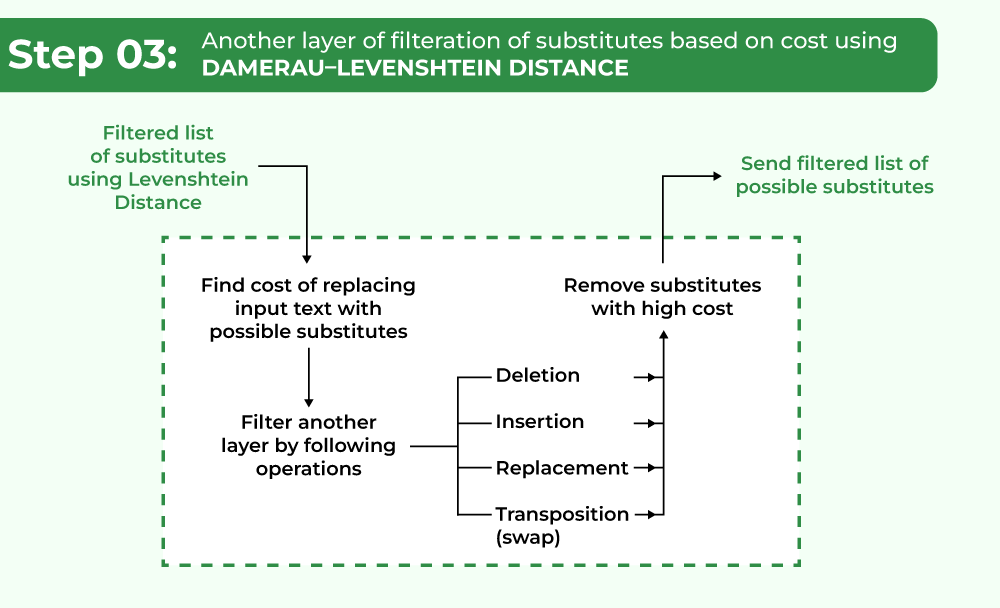Damerau–Levenshtein distance filtration layer based on cost

The Damerau–Levenshtein distance differs from the classical Levenshtein distance by including transposition operation among its allowable operations in addition to the three single-character edit operations (insertions, deletions, and substitutions).

After performing the above operation on the words the cost for all four operations is calculated and the minimum cost operation is chosen.

## 4. Filtering non-related substitutes using Hidden Markov model:

A Hidden Markov Model can describe a situation where events occur but cannot be accurately observed. If the
relationship between the observed info and the hidden info is known, the hidden info can be deduced with some likelihood of success. This can be done to estimate hidden values or predict future hidden values. In this case, the “hidden” info is what the user meant to type, and the “observed” info is what they did type.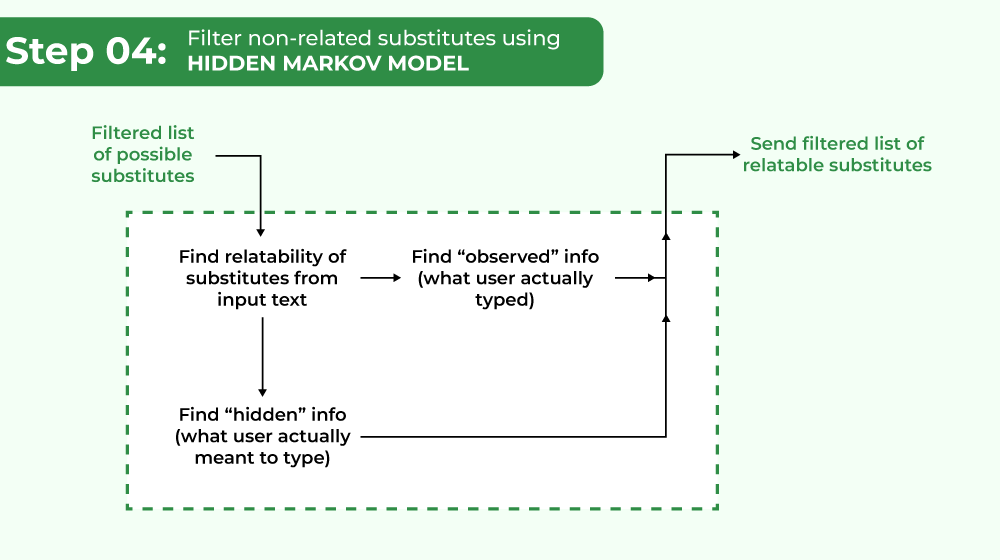Filtering non-related substitutes using Hidden Markov model

It has been recognized that a certain percentage of the time, the user will tap a neighboring key instead of the desired key. For this algorithm to succeed, the user would have to type the correct key at least 60% of the time. The algorithm works on probabilities, which give a variety of probable meanings.

Examples:

2. Hsppy: Happy, hoppy, hippy

## 5. Finding best-matched substitutes based on Probability and Statistics:

Statistics and Probability are two powerful tools. What if we can say that the likelihood of something occurring is greater than the other? Suppose we had some statistics showing what I usually eat on Sunny and rainy days. If it is a sunny day, I would eat ice cream and if it is a rainy day, I would eat pudding. Based on this information, I have a higher likelihood of eating ice cream on a sunny day.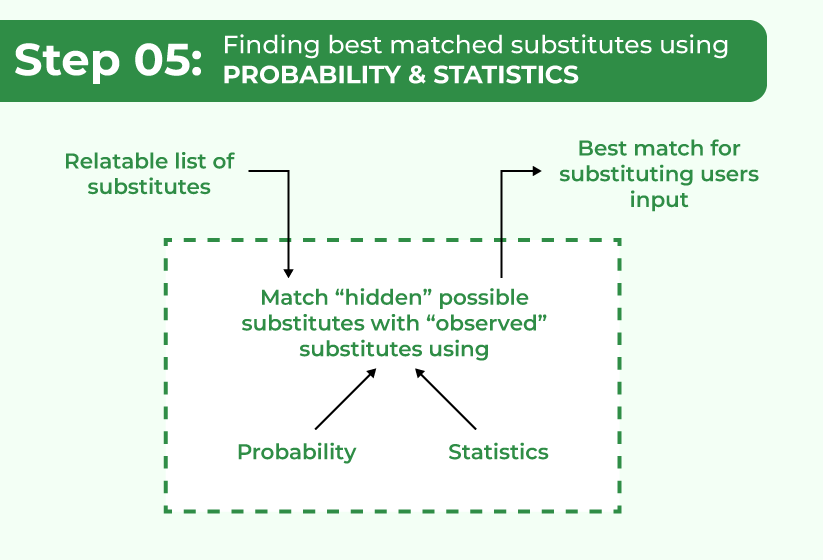Finding best-matched substitutes based on Probability and Statistics

Similarly, The likelihood (probability) of a word’s spelling correction can be determined based on certain information (statistics). Millions of users across the globe misspell words in different languages every day, so the algorithm is programmed to find words that are misspelled in those languages. The algorithm gathers data and makes relevant guesses based on what it considers to be typical.

My Personal Notes arrow_drop_up